Figures for "Efficient trees"

Cameron Browne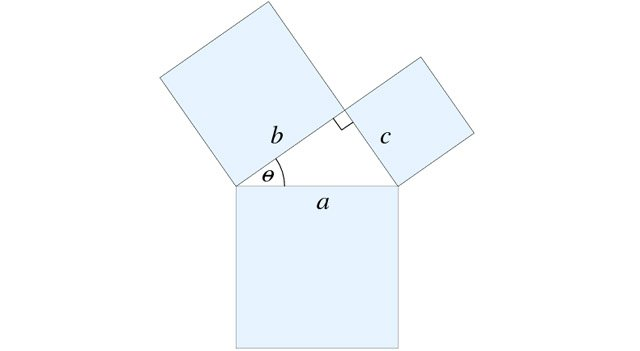Figure 1. Construction based upon the Pythagorean theorem.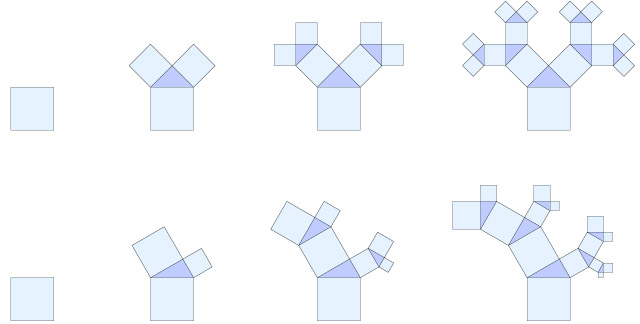Figure 2. First four iterations of the Pythagorean trees P45 (top row) and P30 (bottom row).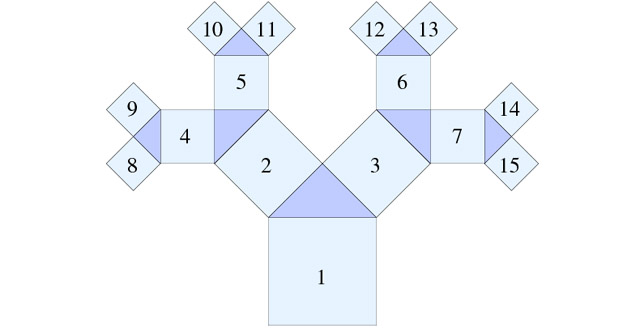Figure 3. Breadth-first growth order of the standard tree.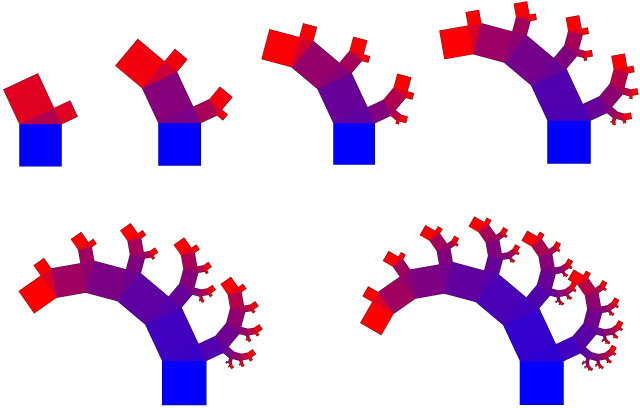Figure 4. Standard tree P25 for i={2, 3, 4, 5, 6, 7}.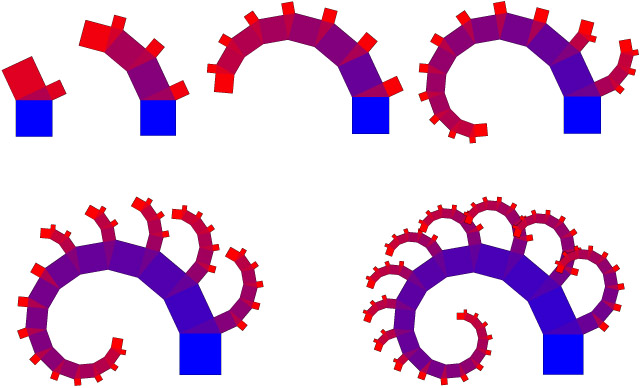Figure 5. Greedy tree P25 for i={2, 3, 4, 5, 6,7}.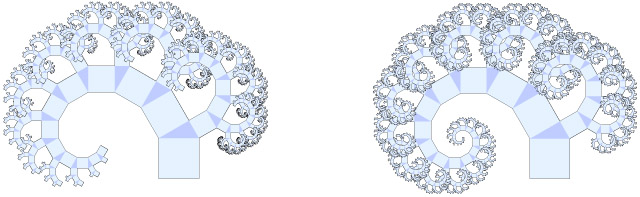Figure 6. Standard and greedy forms of P30 at i=12 (8,191 segments).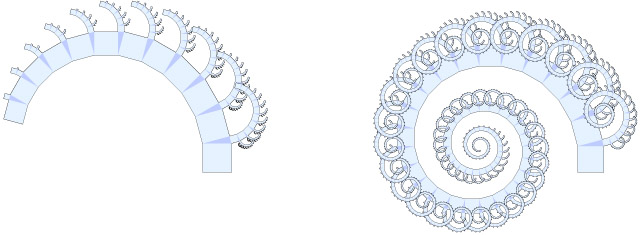Figure 7. Standard and greedy forms of P15 at i=12 (8,191 segments).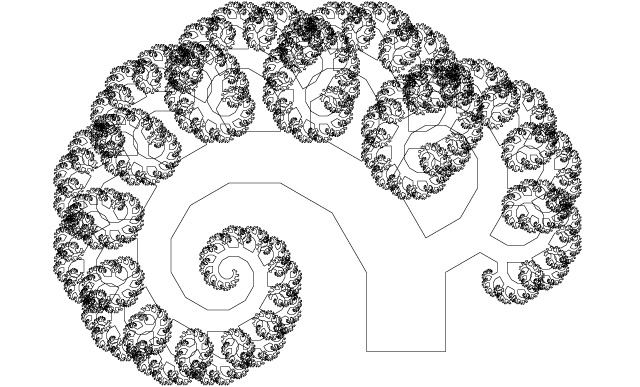Figure 8. Self-overlapping outline of P30 at i=15 (65,535 segments) and w=0.3.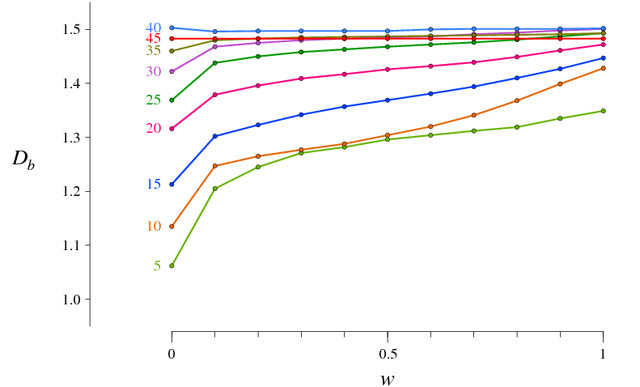Figure 9. Fractal dimension (Db) plotted against weighting w for trees P5..45.